MORE IN Signals & Systems
Total marks: --
Total time: --
INSTRUCTIONS
(1) Assume appropriate data and state your reasons
(2) Marks are given to the right of every question
(3) Draw neat diagrams wherever necessary

1 (a) If x(t) and y(t) are as shown in Fig, Q1(a), sketch x(1-t).y(t/2).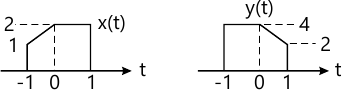6 M
1 (b) If x(n) is as shown in Fig 1(b), find the energy of the signal x(2n-1).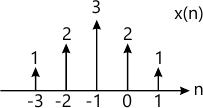4 M
1 (c) Find whether the system represented by y(t)=x(t/2) is linear, Ti. Causal substantiate your answers.
5 M
1 (d) Express x(t) in terms of g(t) if x(t) and g(t) are as shown in Fig. Q1(d).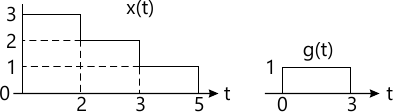5 M

2 (a) Perform the convolution of the two signals.
Using the formula $y(t) = \int^\infty_{-\infty} h(\tau}x(t-\tau) d\tau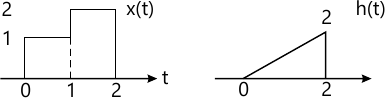10 M 2 (b) Perform the convolution of two finite sequence using graphical method only: \[ x(n)= \left \{ -1, 1, \underset {\uparrow}0, 1 -1\right \} \ \ \ h(n) = \left \{ 1, \underset {\uparrow}2, 3 \right \}$
10 M

3 (a) Find natural, forced and total response for the differential equation:
y''(t)+4y'(t) + 4y(t)=e-2t u(t), assume y(0)=1, y'(0)=0.
9 M
3 (b) Find whether LTI system given by: y(n)=2x(n+2)+3x(n)+x(n-1) is causal. Justify your answer.
4 M
3 (c) Draw DF-I and DF-II implementations for the differential equation: $\dfrac {d^2 y(t)}{dt^2} + \dfrac {5dy(t)}{dt}+ 4y(t) + \dfrac {dx(t)}{dt}$
7 M

4 (a) Consider the periodic waveform x(t)=4+2 cos 3t+3 sin 4t
i) Find period 'T'
ii) What is the total average power
iii) Find the complex Fourier coefficients
iv) Using Parseval's theorem, find the power spectrum
v) Show that total average power using Parseval's theorem is same as obtained in part (2) of the question.
12 M
4 (b) Find FT of the following: i) x(t)=δ(t-2)
ii) x(t)=e-0t u(t).
iii)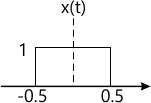8 M

5 (a) Find inverse FT of $x(\omega) = \dfrac {j\omega} {j\omega + 2)^2 }$
6 M
5 (b) Find the DTFT of the rectangular pulse sequence shown in Fig, Q5(b).
Also Plot x(Ω).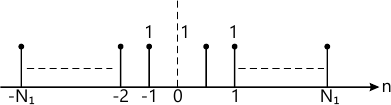10 M
5 (c) Find DTFT of x(n)-δ(4-2n).
4 M

6 (a) State sampling theorem. What is aliasing explain?
4 M
6 (b) Specify the Nyquist rate and Nyquist intervals for each of the following signals:
i) g(t)=sinc2 (200 t)
ii) g(t)=sin c (200 t) - sin c2 (200 t).
6 M
6 (c) Find the FT of the signum function, x(t)=sgn(t). Also draw the amplitude and phase spectra.
10 M

7 (a) State and prove the following properties of Z transform:
i) Multiplication by a R amp
ii) Convolution in time domain.
6 M
7 (b) Find Z-transform of the following and specify its RoC. $x(n)=\sin \left ( \dfrac {\pi}{4}n - \dfrac {-\pi}{2} \right ) u (n-2) ; \\ x(n)= \left ( \dfrac {2}{3} \right )^n u(n)*2^n u (-n-3)$
8 M
7 (c) Find IZT, $if \ \dfrac { \left ( \frac {1}{4} \right )z^{-1}}{\left (1-\frac {1}{2}z^{-1} \right ) \left ( 1- \frac {1}{4} z^{-1} \right )}$ for all possible RoC's.
6 M

8 (a) Solve the difference equation using Z-transform, y(n)=y(n-1)-y(n-2)+2; n≥0 with initial conditions: y(-2)=1, y(-1)=2.
8 M
8 (b) Consider the system described by difference equation.
y(n) - 2y(n-1) + 2y(n-2) = x(n) + 1/2 x(n-1)
i) find system function H(z)
ii) find the stability of the system
iii) find h(n) of the system.
8 M
8 (c) Perform IZT using long division method: $x(z) = - \dfrac {z} {z-a}$ RoC |z|>|a|.
4 M

More question papers from Signals & Systems